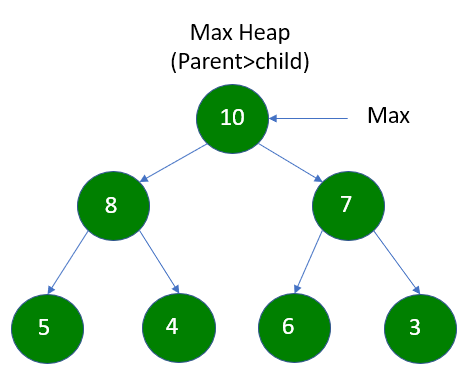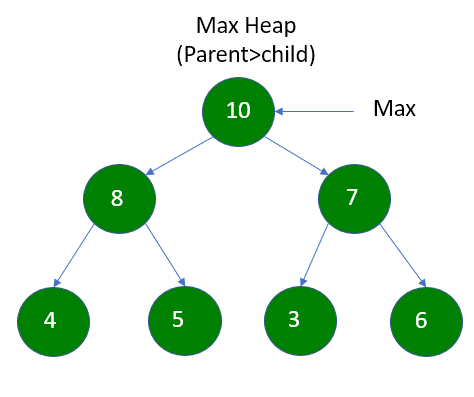Open in App
Not now

# When building a Heap, is the structure of Heap unique?

• Last Updated : 11 Jan, 2023

## What is Heap?

A heap is a tree based data structure where the tree is a complete binary tree that maintains the property that either the children of a node are less than itself (max heap) or the children are greater than the node (min heap).

## Properties of Heap:

Structural Property: This property states that it should be A Complete Binary Tree

For example:Max Heap

Ordering Property: The heap should follow either Max-heap or Min-heap Property.

• If it is Min-heap, the parent node should be less than the child node and
• In the case of a Max-heap, the parent node should be greater than the child node.

These rules should be followed at each level but the order of bottom child nodes can change we can understand it with an example(We are taking Max heap as an example):Max Heap(Figure 1)Max Heap(Figure 2)

## Is the structure of a Heap unique?

From the above two examples, we can see that the heap follows the Max-heap property. And here we can also see that in the first example the child nodes at the bottom level are (5 and 4), (6 and 3) but in the second nodes the child node at the bottom level are (4 and 5), (3 and 6). The place is changed of nodes at the bottom of the heap but it satisfies the condition of Max-heap.

• The order of child or leaf nodes is not fixed, So there will be 4! = 24 valid arrangements possible.
• So which arrangement we get is dependent on the order of insertions and removals or insertion and removal algorithms. Or in the case of making a heap from an array(O(n) case), the order of the array when we start.

There are actually two common types of heaps: min heaps and max heaps.

In a min heap, the parent nodes have values that are less than or equal to the values of their children. In a max heap, the parent nodes have values that are greater than or equal to the values of their children.

The structure of a heap is not unique, because there can be multiple valid heap structures for a given set of values. However, the property of being a min heap or a max heap is unique for a given set of values.

For example, consider the following set of values: [5, 8, 9, 10, 11, 13]. This set of values could be arranged in a min heap as follows:

5
/ \
8  10
/ \   \
9  11  13
This same set of values could also be arranged in a max heap as follows:

13
/ \
11   9
/ \   /
5   8 10
Both of these structures are valid heap structures, but one is a min heap and the other is a max heap.

Heaps are complete binary trees, which means that they are filled in level-order, with the exception that the last level may not be completely filled. This means that the tree is always balanced, which is one of the key properties that makes heaps efficient.

Heaps can be implemented using arrays. In this case, the children of the element at index i are at indices 2i+1 and 2i+2, and the parent of the element at index i is at index (i-1)/2 (assuming that the array is 0-indexed).

Heaps have a number of useful properties that make them useful in a variety of algorithms. For example:

The minimum (or maximum) element can be found in a constant number of steps (this is the root of the heap).
The heap property can be restored by “bubbling up” or “sinking down” an element that has been added or removed.
Heapsort, a sorting algorithm, can be implemented using a heap.

## Conclusion:

From the above images, we can conclude while building a heap, the structure of the heap is not Unique.

My Personal Notes arrow_drop_up